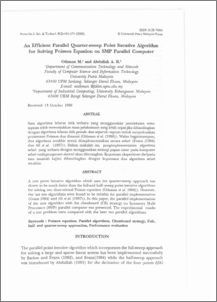# An Efficient Parallel Quarter-sweep Point Iterative Algorithm for Solving Poisson Equation on SMP Parallel Computer

## Citation

M., Othman and A. R., Abdullah (2000) An Efficient Parallel Quarter-sweep Point Iterative Algorithm for Solving Poisson Equation on SMP Parallel Computer. Pertanika Journal of Science & Technology, 8 (2). pp. 161-174. ISSN 0128-7680

## Abstract

A new point iterative algorithm which uses the quarter-sweep approach was shown to be much faster than the full-and half- sweep point iterative algorithms for solving two dimensional Poison equation (Othman el at. 1998». However, the last two algorithms were found to be suitable for parallel implementation (Evans 1984) and Ali el at. (1997». In this paper, the parallel implementation of the new algorithm with the chessboard (CB) strategy on Symmetry Multi Processors (SMP) parallel computer was presented. The experimental results of a test problem were compared with the later two parallel algorithms.Preview
PDF
An_Efficient_Parallel_Quarter-sweep_Point_Iterative_Algorithm.pdfView Item